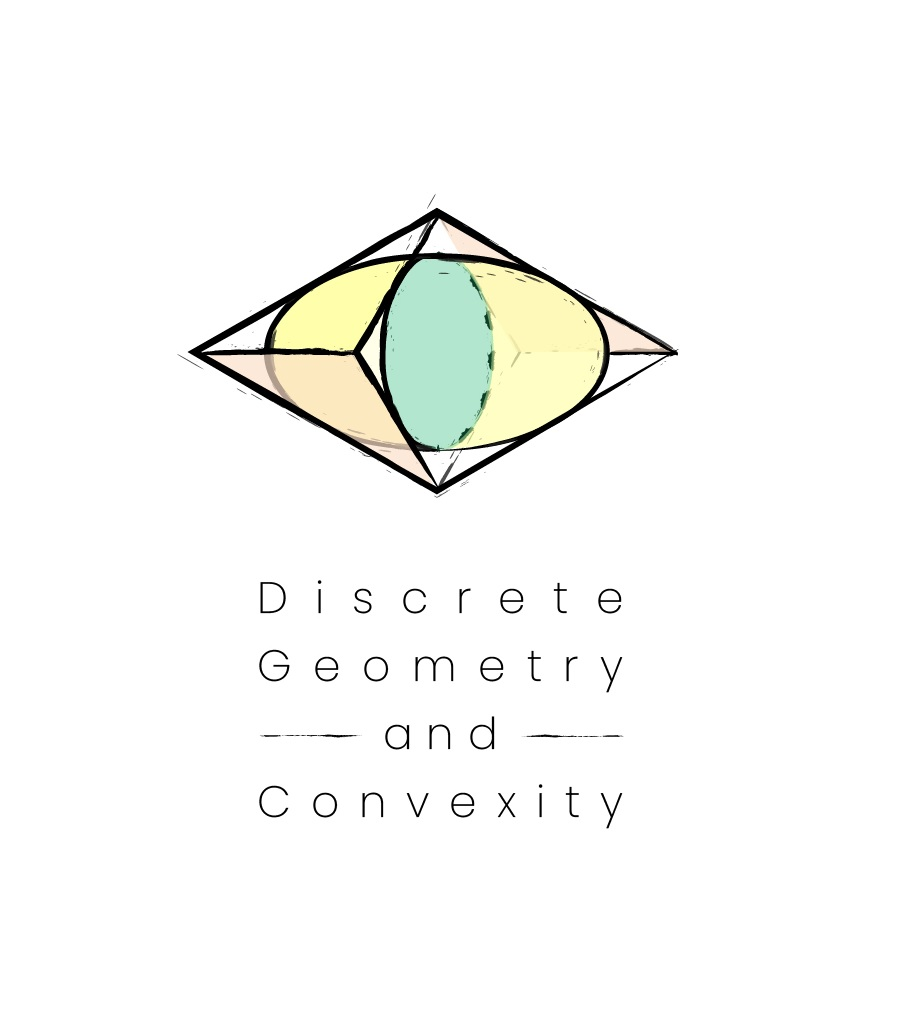x

# Erdős Center Seminar

09.12.2023. - 01.16.2024.
Every Tuesday at 10:00 in the Tondos room of the Rényi Institute.

### DescriptionIn the Fall of 2023 the Erdős Center of the Rényi Institute organizes a special semester on Discrete Geometry and Convexity. In the framework of this program, we will run a special seminar, attended by the participants and all members of our institute interested in the subject. The talks are typically on Tuesday 10-12 am, in the Tondos room of the Rényi Institute.

September 11, 2023, 10am(CET) Note that this talk is on Monday!!

Peter van Hintum and Marius Tiba:  Sharp stability for the Brunn-Minkowski inequality for arbitrary sets

Abstract: The Brunn-Minkowski inequality states that for (open) sets $A$ and $B$ in $R^d$, we have $|A+B|^{1/d} \geq |A|^{1/d}+|B|^{1/d}$. Equality holds if and only if $A$ and $B$ are convex and homothetic sets in $R^d$. In this talk, we present a sharp stability result for the Brunn-Minkowski inequality, concluding a long line of research on this problem. We show that if we are close to equality in the Brunn-Minkowski inequality, then $A$ and $B$ are close to being homothetic and convex, establishing the exact dependency between the three notions of closeness. This is based on joint work with Alessio Figalli, Peter van Hintum, and Marius Tiba.

September 19, 2023, 10am(CET)

Ji Zeng: Disjoint faces in simple topological graphs

Abstract: A topological graph is a graph drawn in the plane such that its vertices are represented by points, and its edges are represented by non-self-intersecting curves connecting the corresponding points. A topological graph is simple if any two of its edges intersect at most once, either at a common endpoint or at a proper crossing point. A k-face generated by a topological graph is the open bounded region enclosed by the edges of a non-self-intersecting $k$-cycle.

We prove that every $n$-vertex complete simple topological graph generates at least $\Omega(n)$ pairwise disjoint 4-faces. This improves upon a recent result by Hubard and Suk. As an immediate corollary, every $n$-vertex complete simple topological graph drawn in the unit square generates a 4-face with area at most $O(1/n)$. This can be seen as a topological variant of Heilbronn's problem for 4-faces. We construct examples showing that our result is asymptotically tight. We also discuss the similar problem for $k$-faces with arbitrary $k>=3$.

September 26, 2023, 10am(CET)

Arseniy Sagdeev: Infinite sets in (non-)Euclidean Ramsey Theory.

Abstract: A classic result in Euclidean Ramsey Theory states that for every infinite set of points $M$, there exists a two-coloring of the Euclidean space (of an arbitrary dimension) such that no isometric copy of $M$ is monochromatic. We will see that the direct analogue of this result also holds in $\ell_p$ planes for all real $p>1$, but fails for all polygonal norms. In case $p=\infty$, we will even show that there exists a single infinite set $M$ such that some of its isometric copies are monochromatic whenever an $n$-dimensional space is colored in less than $\log(n)$ colors.

October 3, 2023, 10am(CET)

Travis Dillon: Peeling the grid

Abstract: To peel a finite point set in Euclidean space, remove the vertices of its convex hull. The number of times a point set must be peeled to remove all of its vertices is called the layer number of the set. After outlining previous work on random point sets and "evenly distributed" point sets, I will turn to the grid ${1,2,...,n}^d$, whose layer number remains unknown. The central results of this talk are two short proofs that significantly improve the bounds for the layer number of grids. We show as a consequence that the layer number of grids is linear in $d$.

October 17, 2023, 10am(CET)

Zichao Dong: Convex polytopes in non-elongated point sets in $\mathbb{R}^d$

Abstract: For any finite point set $P \subset \mathbb{R}^d$, we denote by $\text{diam}(P)$ the ratio of the largest to the smallest distances between pairs of points in $P$. Let $c_{d, \alpha}(n)$ be the largest integer $c$ such that any $n$-point set $P \subset \mathbb{R}^d$ in general position, satisfying $\text{diam}(P) < \alpha\sqrt[d]{n}$ (informally speaking, `non-elongated'), contains a convex $c$-polytope. Valtr proved that $c_{2, \alpha}(n) \approx \sqrt{n}$, which is asymptotically tight in the plane. We generalize the results by establishing $c_{d, \alpha}(n) \approx n^{\frac{d-1}{d+1}}$. Along the way we generalize the definitions and analysis of convex cups and caps to higher dimensions, which may be of independent interest. Joint work with Boris Bukh.

October 24, 2023, 10am(CET)

Boris Aronov: TBD

Gábor Damásdi

János Pach

Ji Zeng

Arsenii Sagdeev

Peter van Hintum

Marius Tiba

Travis Dillon

Zichao Dong

Boris Aronov

### Participants

For everyone interested.